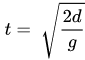# Is it possible to calculate how long a part would take to fall down to the floor

Exactly as the title states, i want to use this for an anti cheat and i cant seem to find an accurate one myself.

1 Like

With raycast calculate the length between the leg and hitpart since its g(t^2)/2 = S if it doesnt have starter velocity if it has then the formula is Vt + g(t^2)/2 = S you know the g is workspace.Gravity you can calculate the t hope this helps

im guessing S is speed, g is gravity and t is time right? and in the other one V is the inital velocity (just to get it clear)

No S is the length you are going in that timewhere:

• t is time
• d is distance (in studs)
• g is rate of gravity, which can be found in game settings I think (in studsper second, default is 196.2s/s)

sooo

``````local distance = 100
local TimeToFall = math.sqrt((2*distance)/196.2))
print(TimeToFall)
``````

oh alright i rearranged it to that aswell i was just confused about s thx